# 978-0123869449 Chapter 4 Part 2

Document Type
Homework Help
Book Title
Authors
Michael F. Modest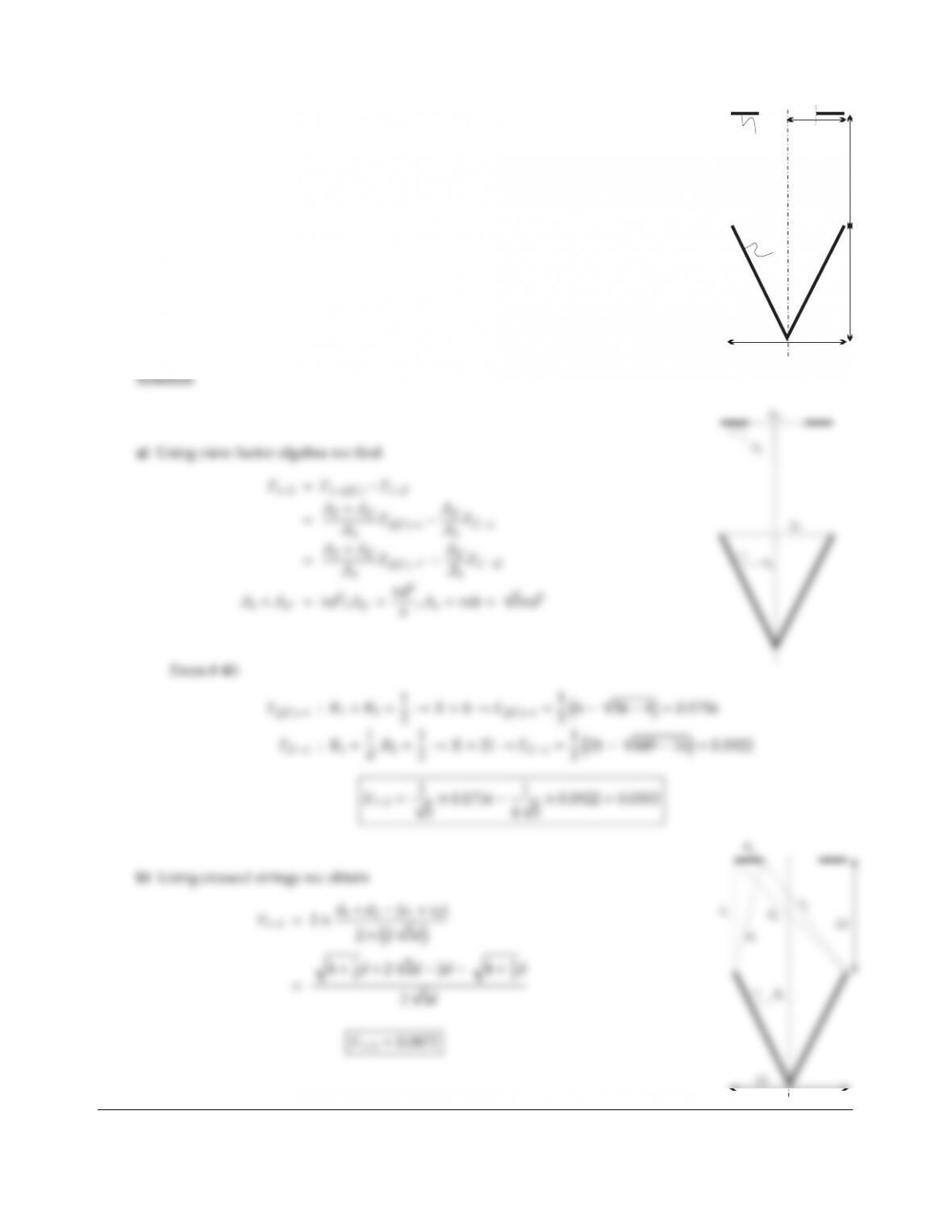CHAPTER 4 83
4.16
2d
2d
A2
A1
2d
d/2 d/2
Consider the configuration shown; determine the view factor F12assuming the
configuration
a) to be axisymmetric (1 conical, 2 a disk with hole), or
b) to be two-dimensional Cartesian (1 is a V-groove, 2 two infinitely long strips).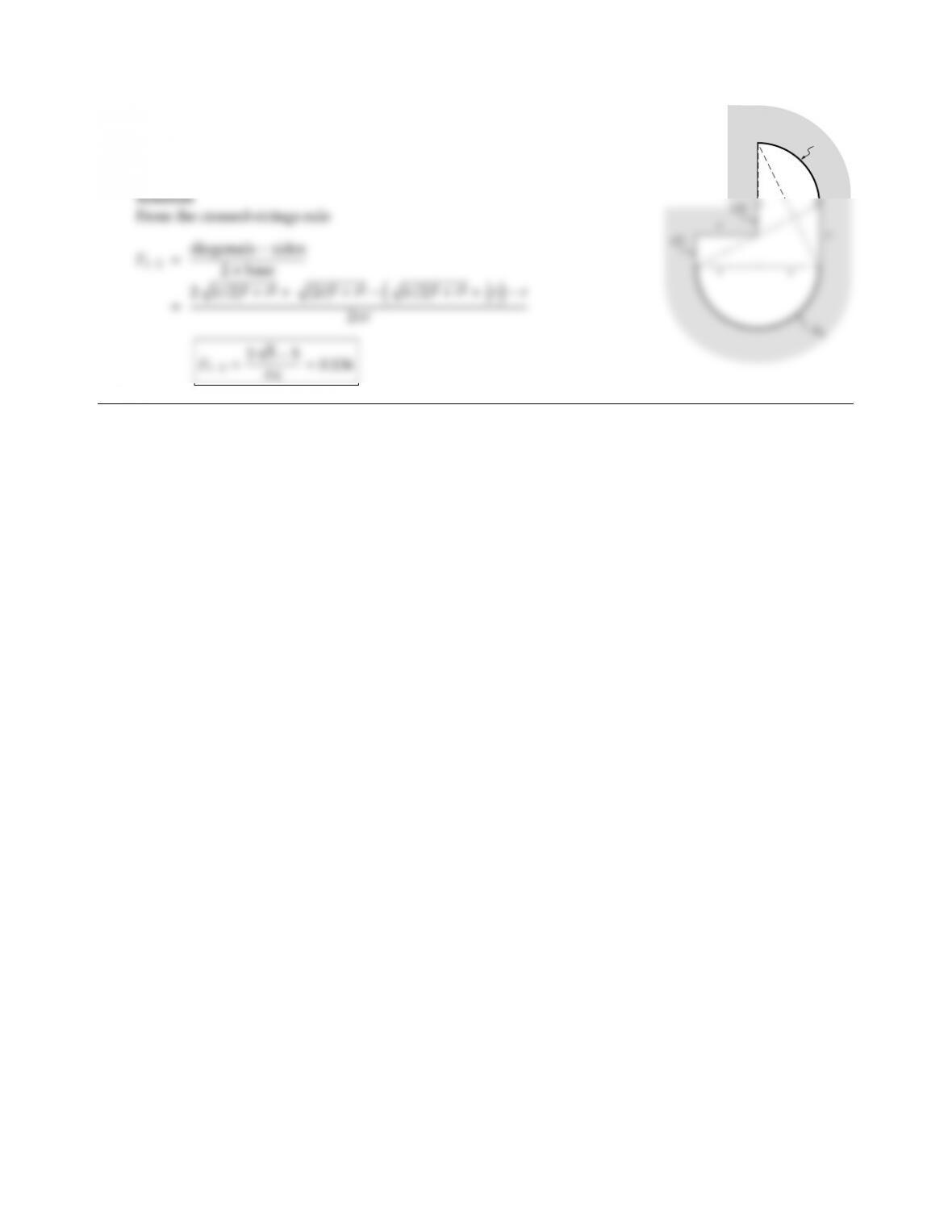4.17
r
A2
Find F12for the configuration shown in the figure (infinitely long perpen-
dicular to paper).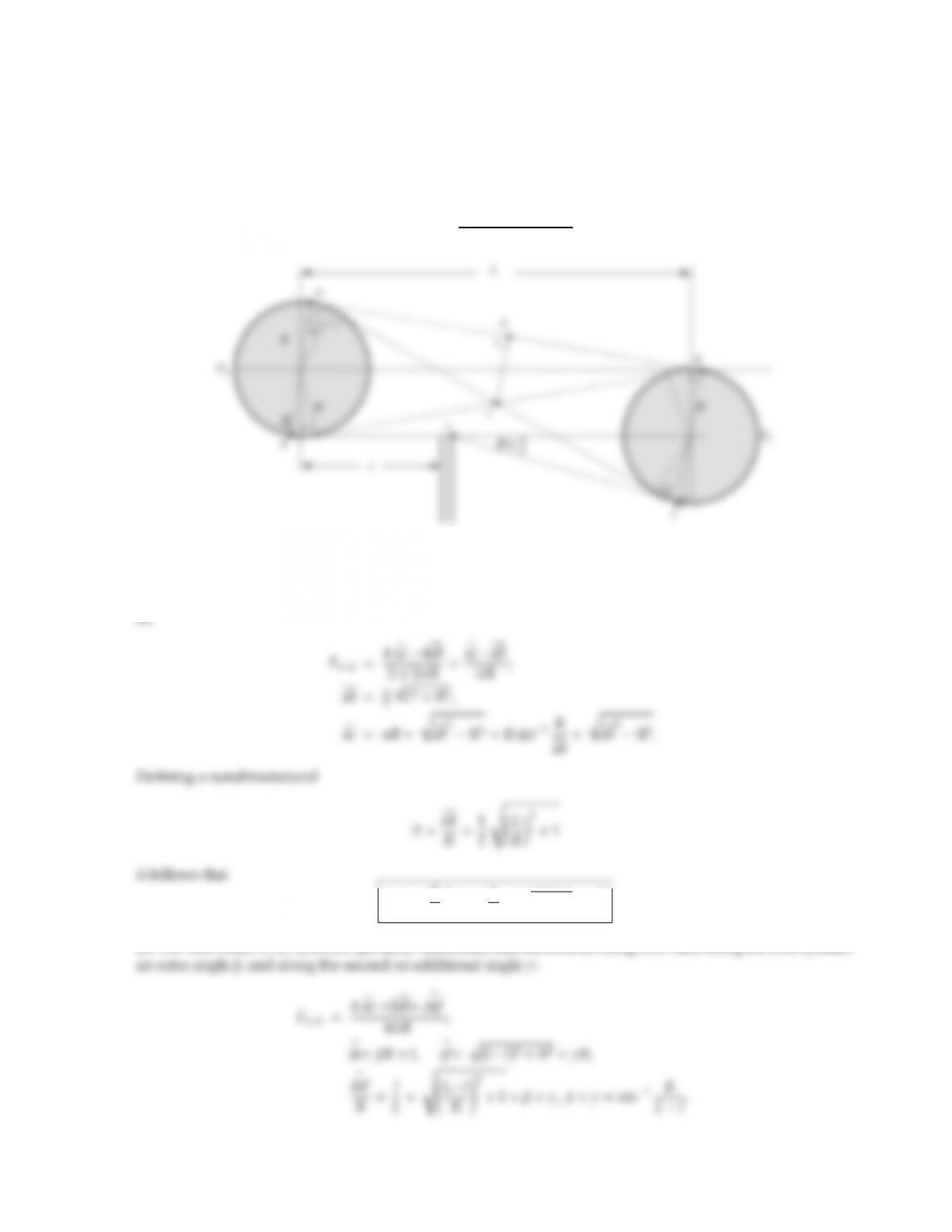CHAPTER 4 85
4.18 Calculate the view factor between two infinitely long cylinders as shown in the figure. If a radiation shield is
placed between them to obstruct partially the view (dashed line), how does the view factor change?
Solution
The view factor is most easily determined by the crossed strings method,
F12=diagonals sides
2×base .
To evaluate the lengths, two “pins” are placed right next to each other on the backside of each cylinder, say
points P1and P2. For both cases, (a) and (b), the strings of diagonals and sides run along the cylinder walls
from P1to aand d, and from P2to fand 1, thus cancelling each other. Therefore:
(a)
F12=1
πsin11
X+X21X
(b) The view from A1to A2is now partially obstructed, and the bottom string now runs along the first cylinder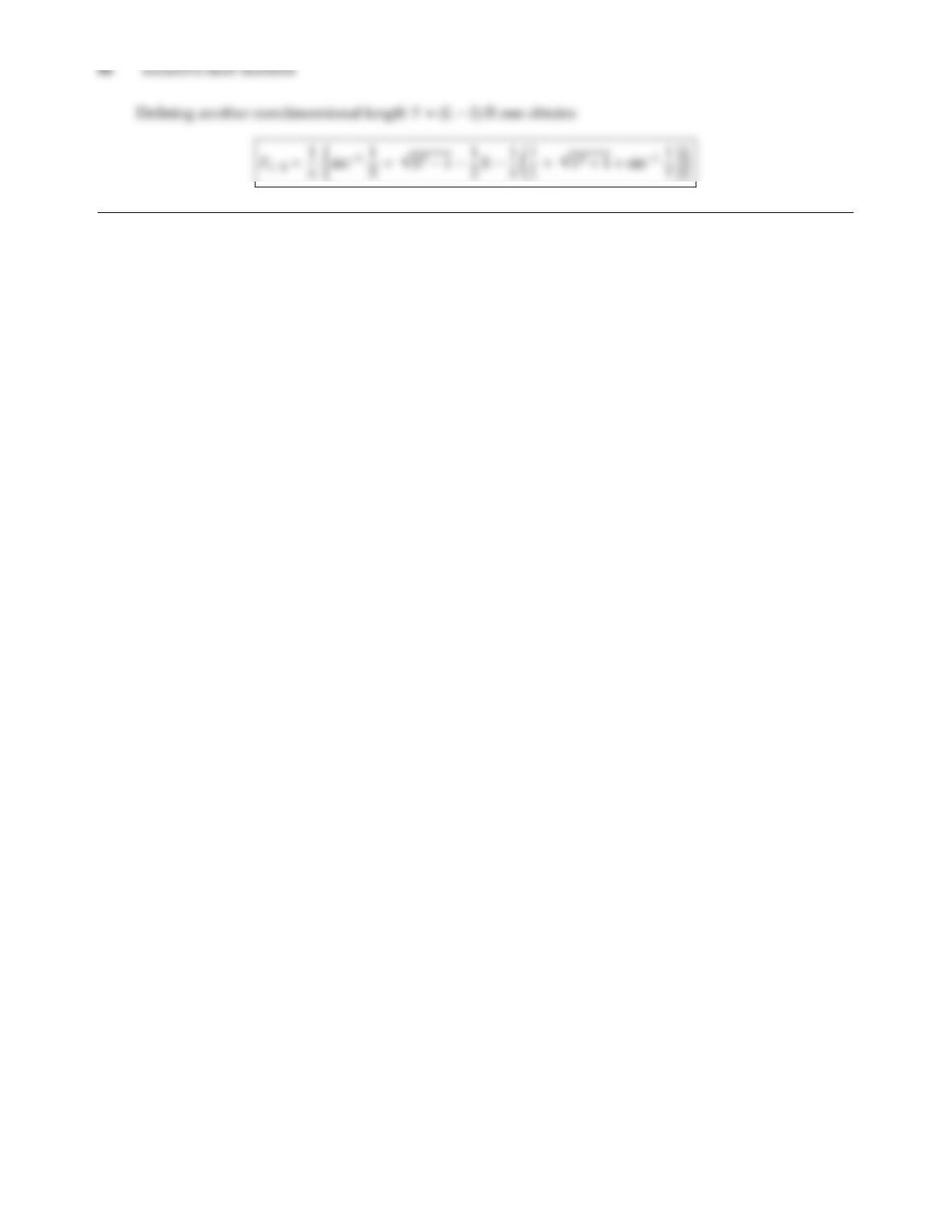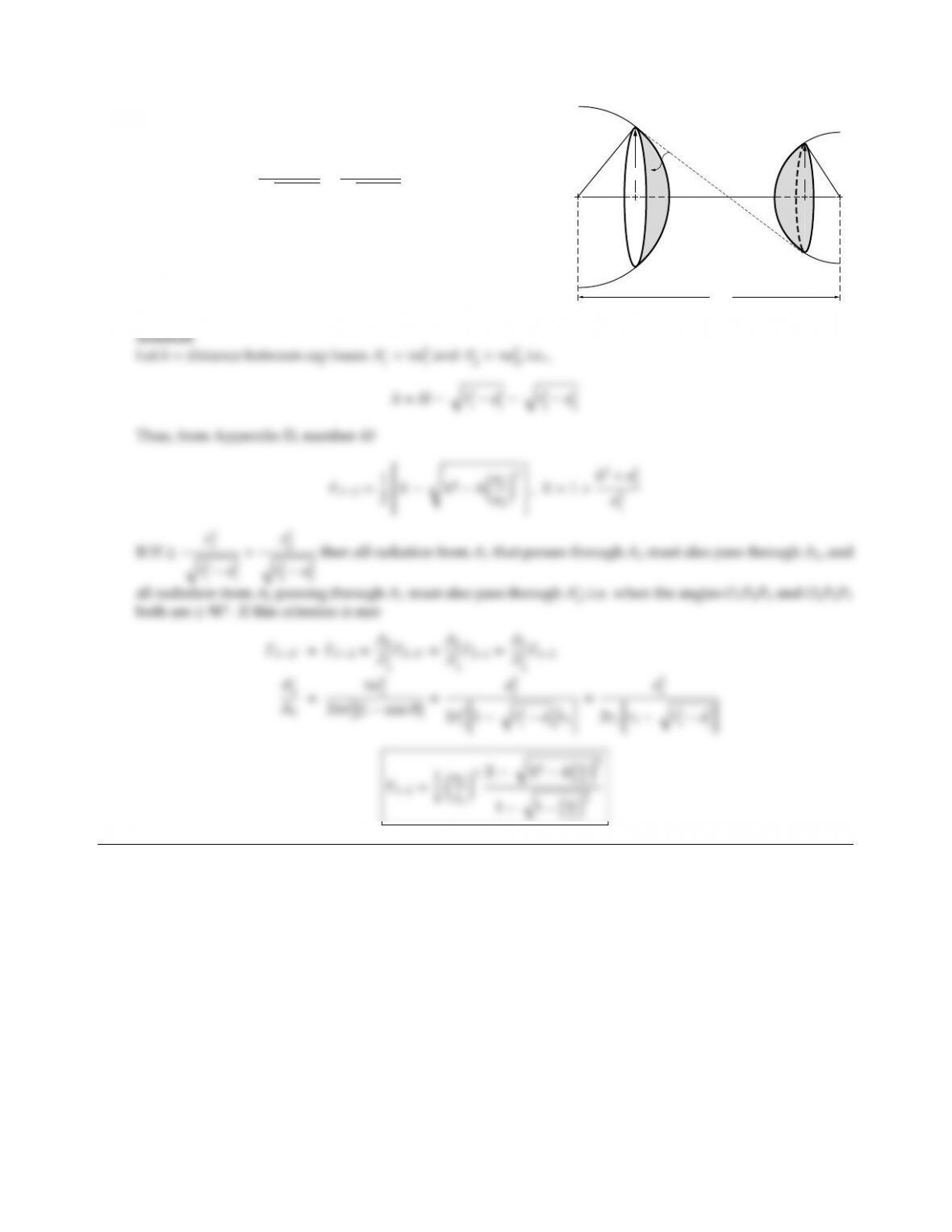CHAPTER 4 87
4.19
H
R1A1R2
a1a2
O1
O2
P1
P2
Find the view factor between spherical caps as shown in
the figure, for the case of
HR2
1
qR2
1a2
1
+R2
2
qR2
2a2
2
,
where H=distance between sphere centers, R=sphere
necessary?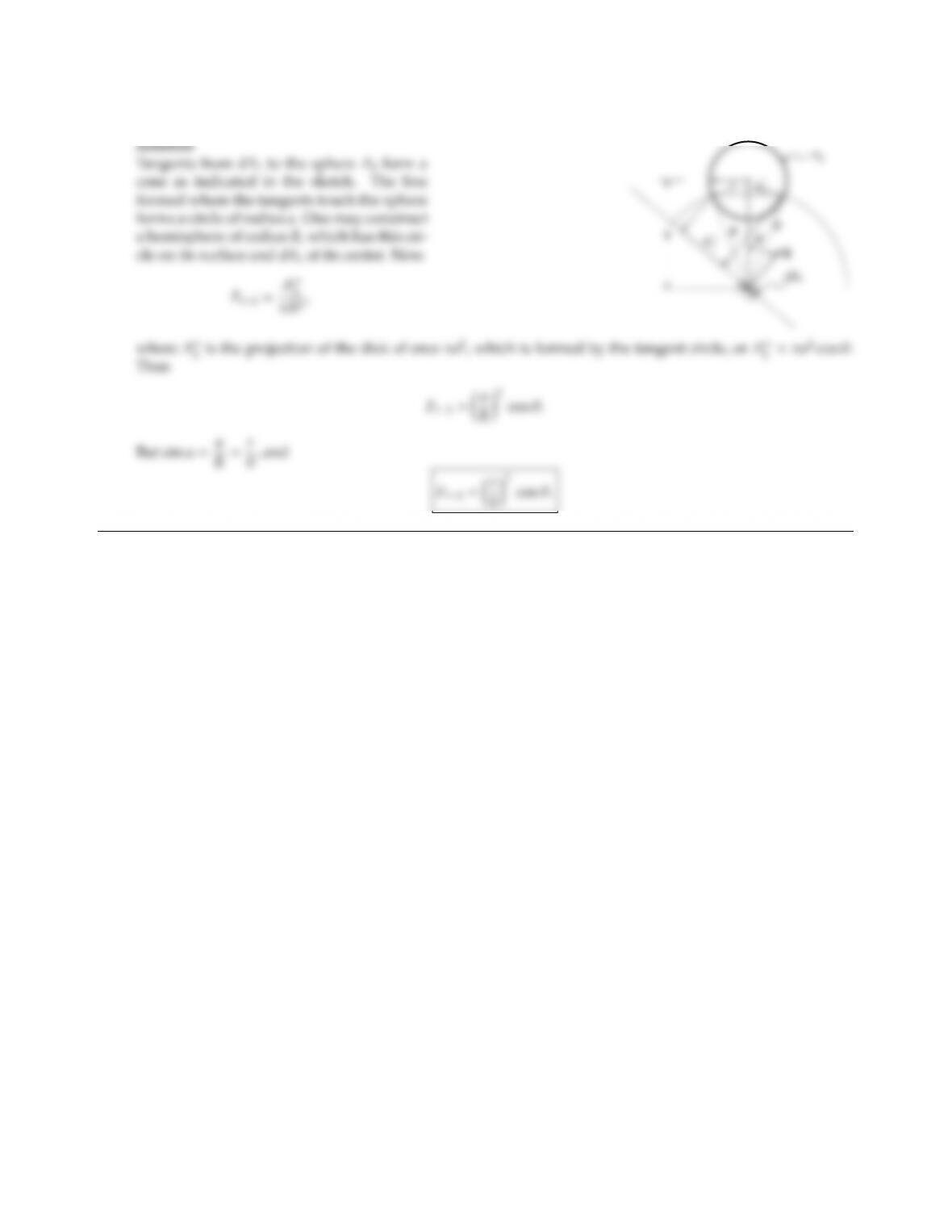4.20 Determine the view factor for Configuration 18 in Appendix D, using the unit sphere method.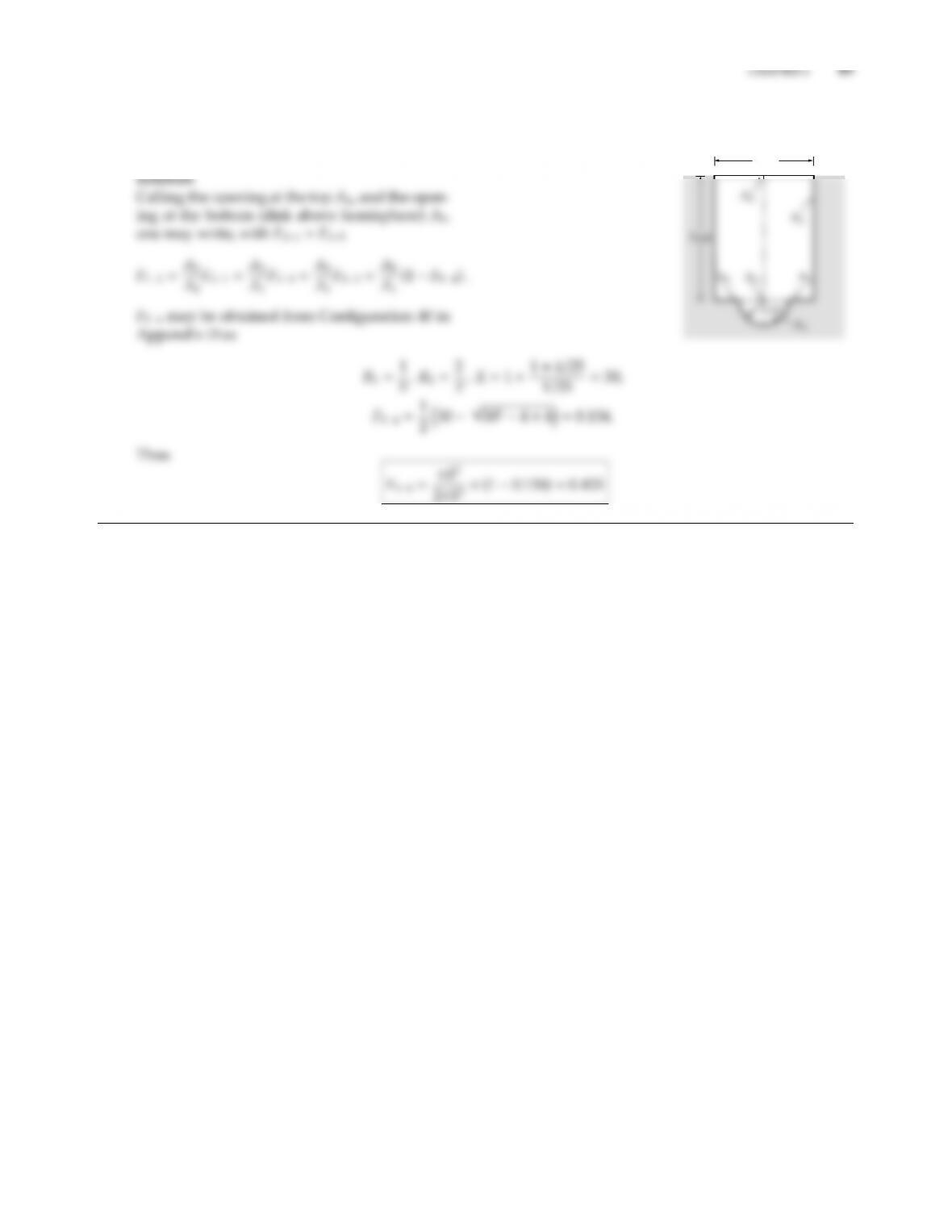4.21 Consider the axisymmetric configuration
shown in the figure. Calculate the view factor
F13.
4cm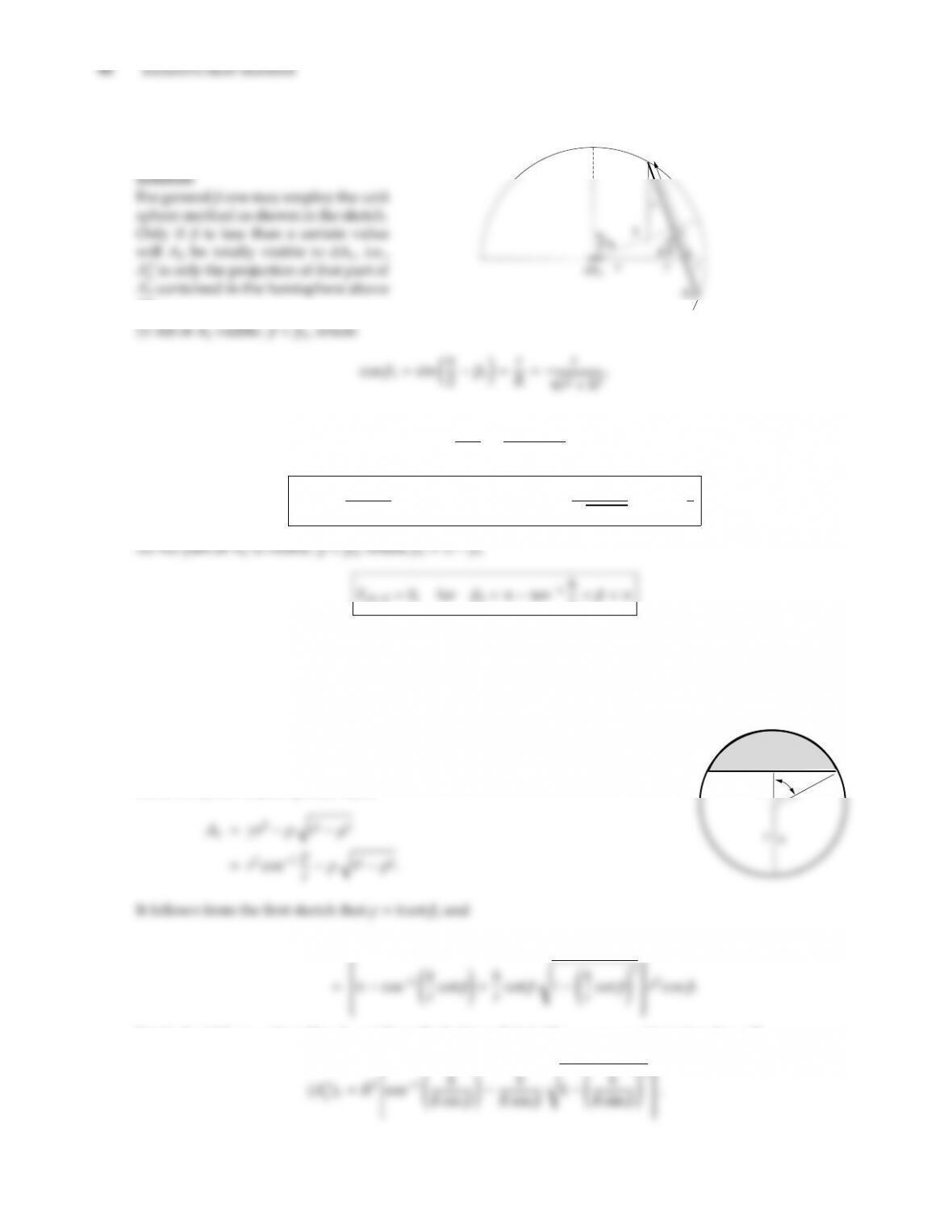4.22 Find Fd12from the infinitesimal area
to the disk as shown in the figure, with
0βπ.
dA1.
Fd12=A′′
2
πR2=πr2cos β
π(r2+h2)
Fd12=r2cos β
r2+h2,for 0 < β < β1=cos1r
r2+h2
=tan1h
r
r< β < π
(iii) A2is partially visible: β1< β < β2.
Recalling the principle of the unit sphere method: Ais the projection of (the visible part of) Aonto the sphere,
and A′′ is the projection of Aonto the bottom disk. Therefore, A′′
2consists of two parts: the projection of the
upper part of the disk (indicated by “y” in the sketch), and a part of the bottom disk (indicated by “z”).
When two disks, such as disk A2and the base disk
containing dA1, intersect each other, the cut is a
straight line as indicated in the sketch on the right
(looking from the bottom of A2towards dA1). The
sliver of A2not visible by dA1,Ac, is
Ac
γ
ρ
(A′′
2)y=(πr2Ac) cos β
Similarly, (A′′
2)zis a sliver like Ac, cut from the bottom disk (with ρ=x+y=h/sin βand r=R),CHAPTER 4 91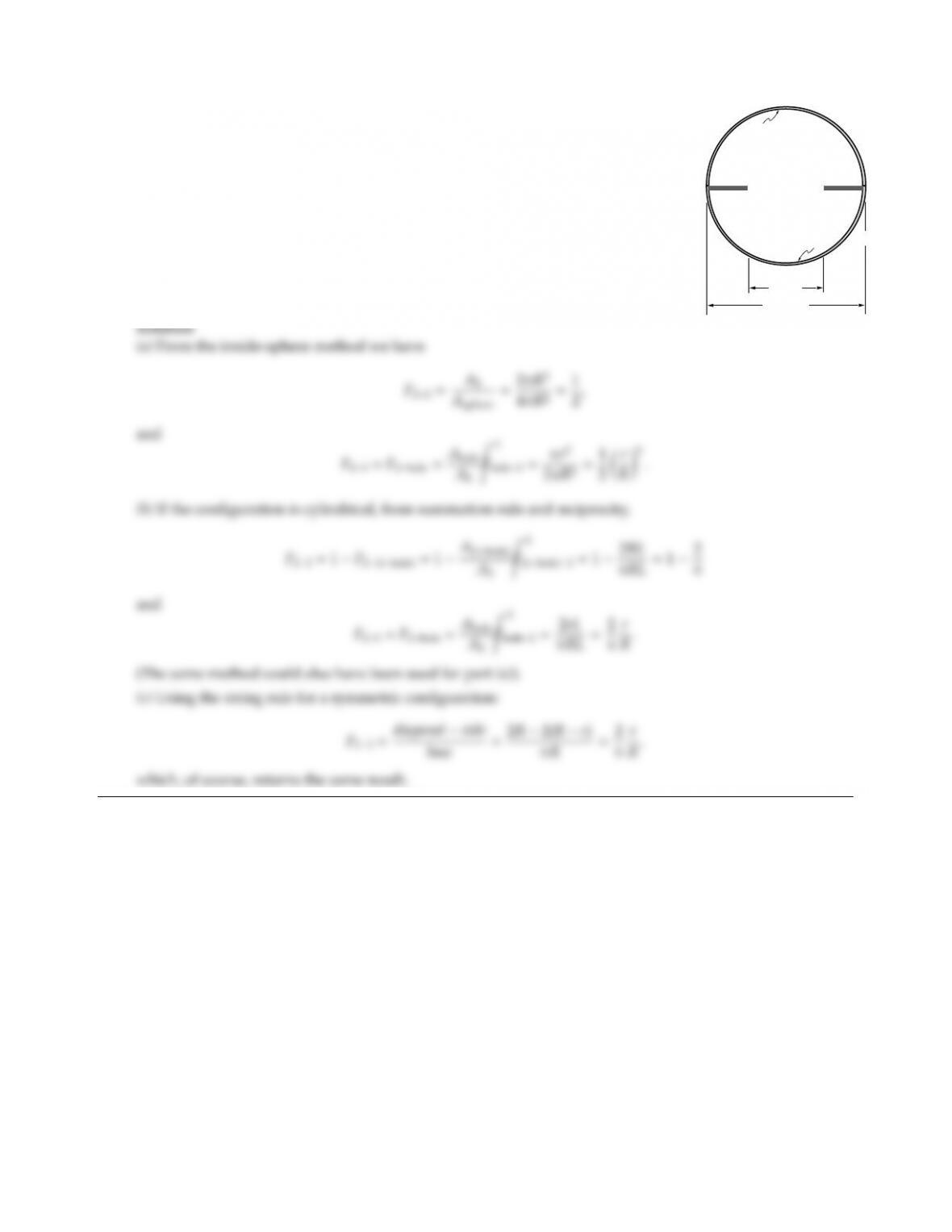4.23
2r
2R
A1b
A1t
A2
A3
Consider the configuration shown (this could be a long cylindrical BBQ with a
center shelf/hole; or an integrating sphere). Determine the view factors F22and
F23assuming the configuration
(a) to be axisymmetric (sphere),
(b) to be two-dimensional Cartesian (cylinder), using view factor algebra,
(c) to be two-dimensional Cartesian (cylinder), using the string rule (F23only).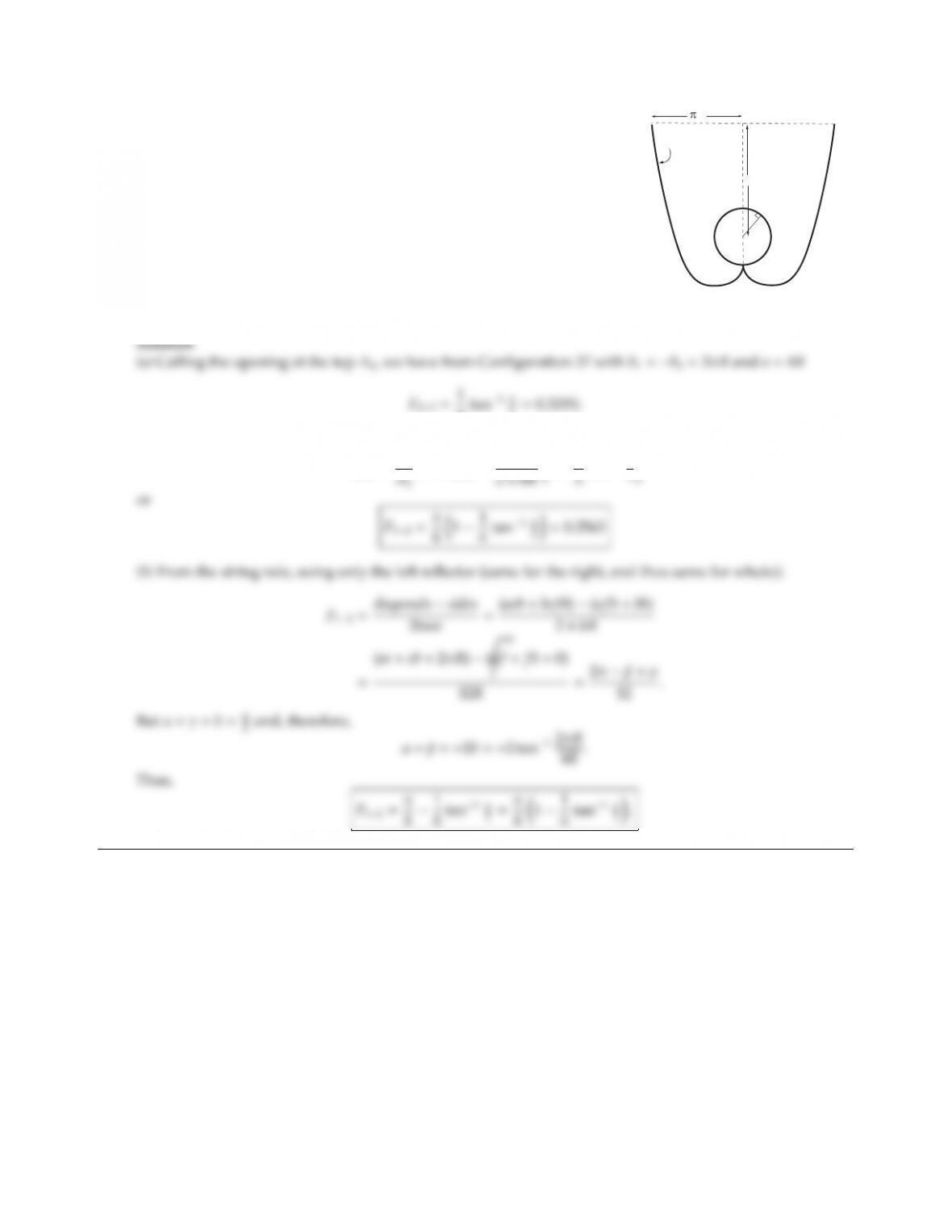CHAPTER 4 93
4.24
R
4R
6R
R
concentrator
oil
tube
In the solar energy laboratory at UC Merced parabolic concentrators are
employed to enhance the absorption of tubular solar collectors as shown
in the sketch. Calculate the view factor from the parabolic concentrator
A1to collecting cylinder A2, using
(a) view factor algebra,
(b) Hottel’s string rule.
πtan1π
2=0.3195;
from view factor algebra
F12=A2
(1 F23)=2πR
2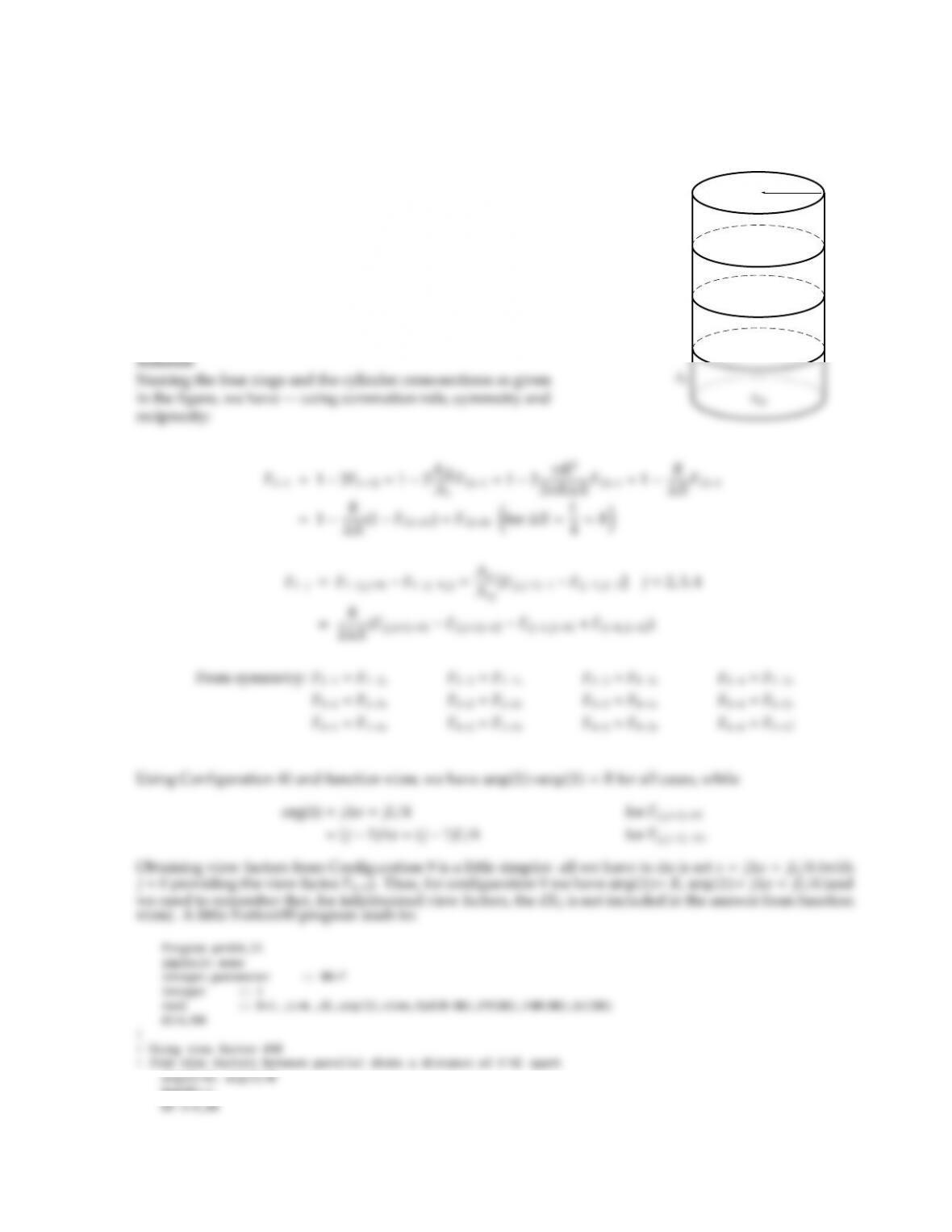4.25 The interior of a right-circular cylinder of length L=4R, where
Ris its radius, is to be broken up into 4 ring elements of equal
width. Determine the view factors between all the ring ele-
ments, using
(a) view factor algebra and the view factors of Configuration
40,
(b) Configuration 9 with the assumption that this formula can
be used for rings of finite widths.
Assess the accuracy of the approximate view factors. What
would be the maximum allowable value for Xto ensure that
all view factors (within a distance of 4R) are accurate to at least
5%? Use program viewfactors or function view in your calcu-
lations.
L
/4
R
A2
A3
A4
A12
A34
A23
A45
Fpd(0)=1.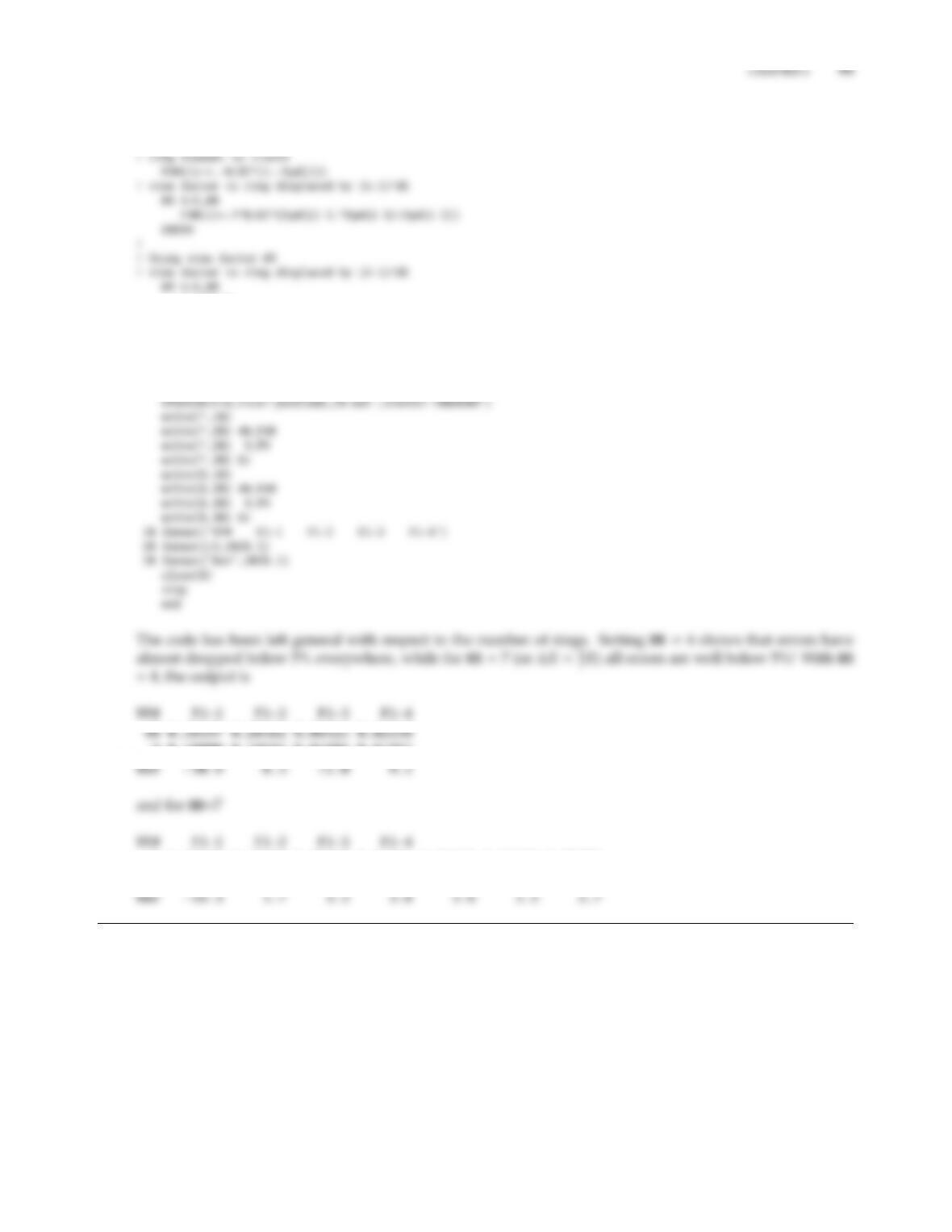arg(1)=i*dl
Fpd(i)=view(40,3,arg)
ENDDO
arg(1)=R
arg(2)=(i-1)*dl
F9(i)=view(9,2,arg)*dl/(2.*R)
Er(i)=100.*(1.-F9(i)/F40(i))
ENDDO
!
! Output
9 0.50000 0.18695 0.05806 0.01997
40 0.24570 0.17398 0.09359 0.04801 0.02498 0.01359 0.00780
9 0.28571 0.17094 0.09053 0.04618 0.02407 0.01316 0.00759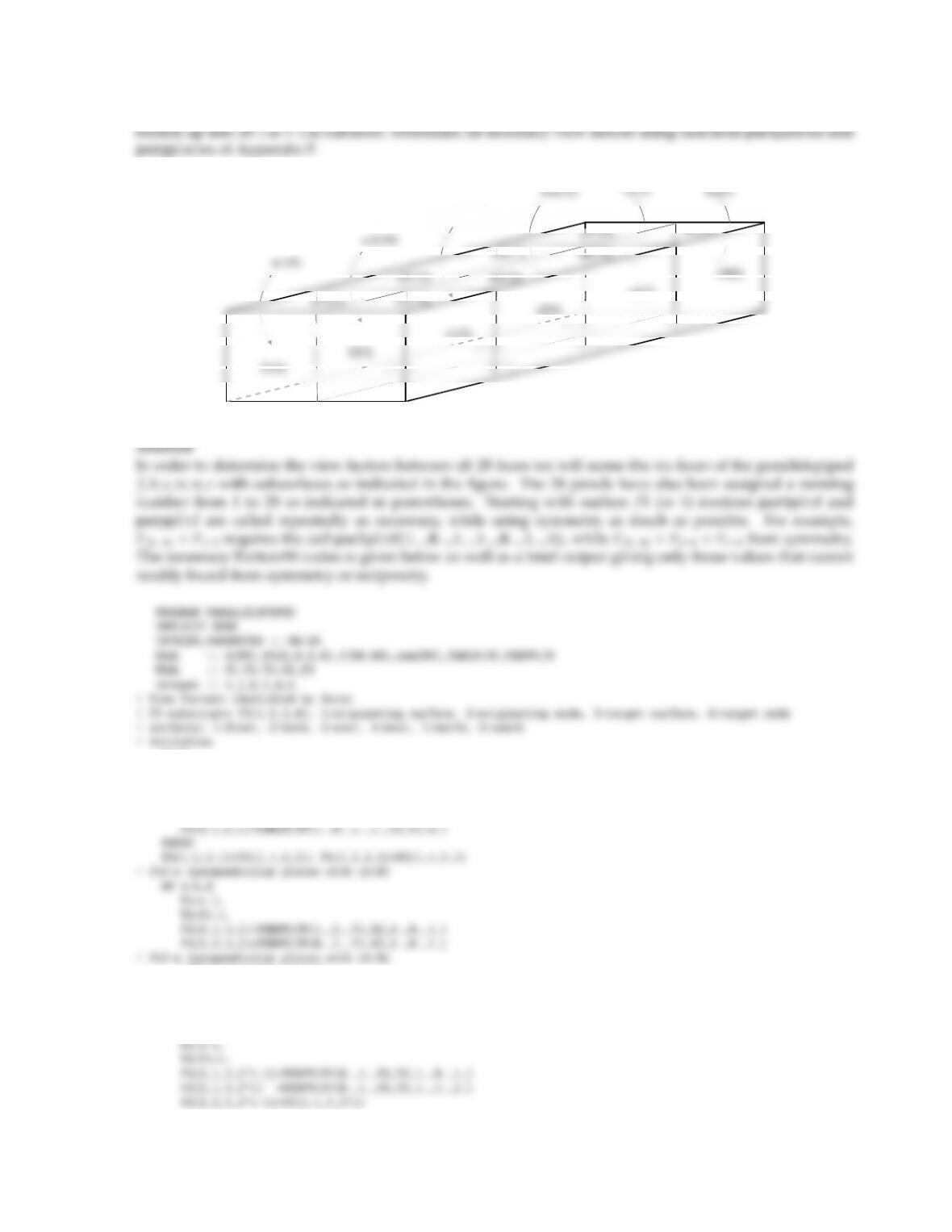4.26 The inside surfaces of a furnace in the shape of a parallelepiped with dimensions 1 m ×2 m ×4 m is to be
e1(5)
e2(6)
e3(7)
e4(6)
w2(10)
w3(11)
s1(21) s2(22)
FS=0.
! FSf-b (parallel plates with x2=0 and c=4)
DO i=1,2
Y2=abs(i-1)
Y3=Y2+1.
FS(1,1,4,i)=FS(1,2,3,i)
FS(1,2,4,i)=FS(1,1,3,i)
ENDDO
! FSf-n (perpendicular plates with x1=0)
DO i=1,4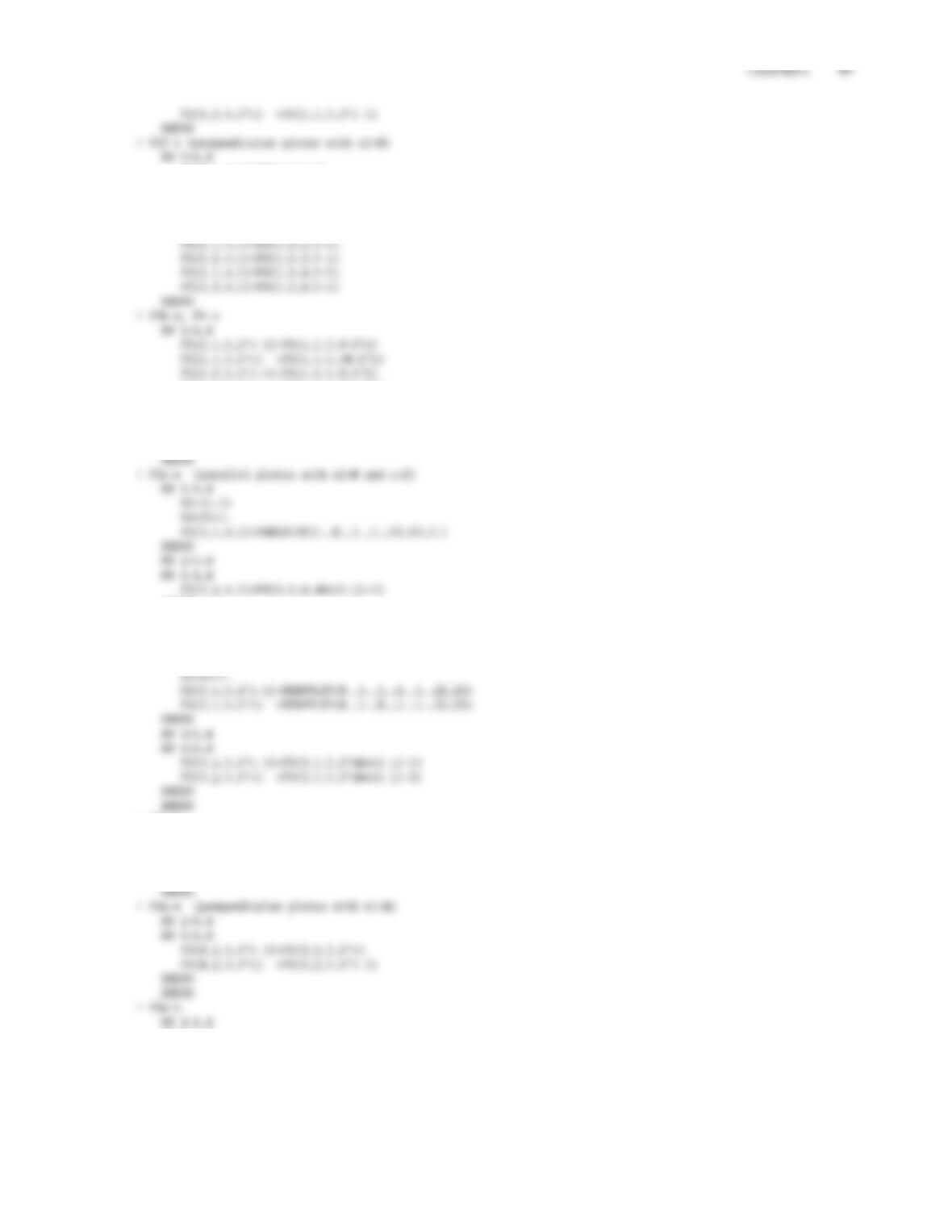FS(1,1,6,i)=FS(1,1,5,i)
FS(1,2,6,i)=FS(1,2,5,i)
ENDDO
! FSb-e, Fb-w
DO i=1,4
FS(2,2,5,2*i) =FS(1,2,5,10-2*i)
FS(2,1,6,2*i-1)=FS(2,1,5,2*i-1)
FS(2,1,6,2*i) =FS(2,1,5,2*i)
FS(2,2,6,2*i-1)=FS(2,2,5,2*i-1)
FS(2,2,6,2*i) =FS(2,2,5,2*i)
ENDDO
ENDDO
! FSe-n (perpendicular plates with x1=0)
DO i=1,4
Z2=i-1.
! FSe-s
DO j=1,4
DO i=1,8
FS(3,j,6,i)=FS(3,j,5,i)
ENDDO
DO i=1,8
FS(4,j,6,i)=FS(4,j,5,i)
ENDDO
ENDDO
! FSn-s (parallel plates with c=1)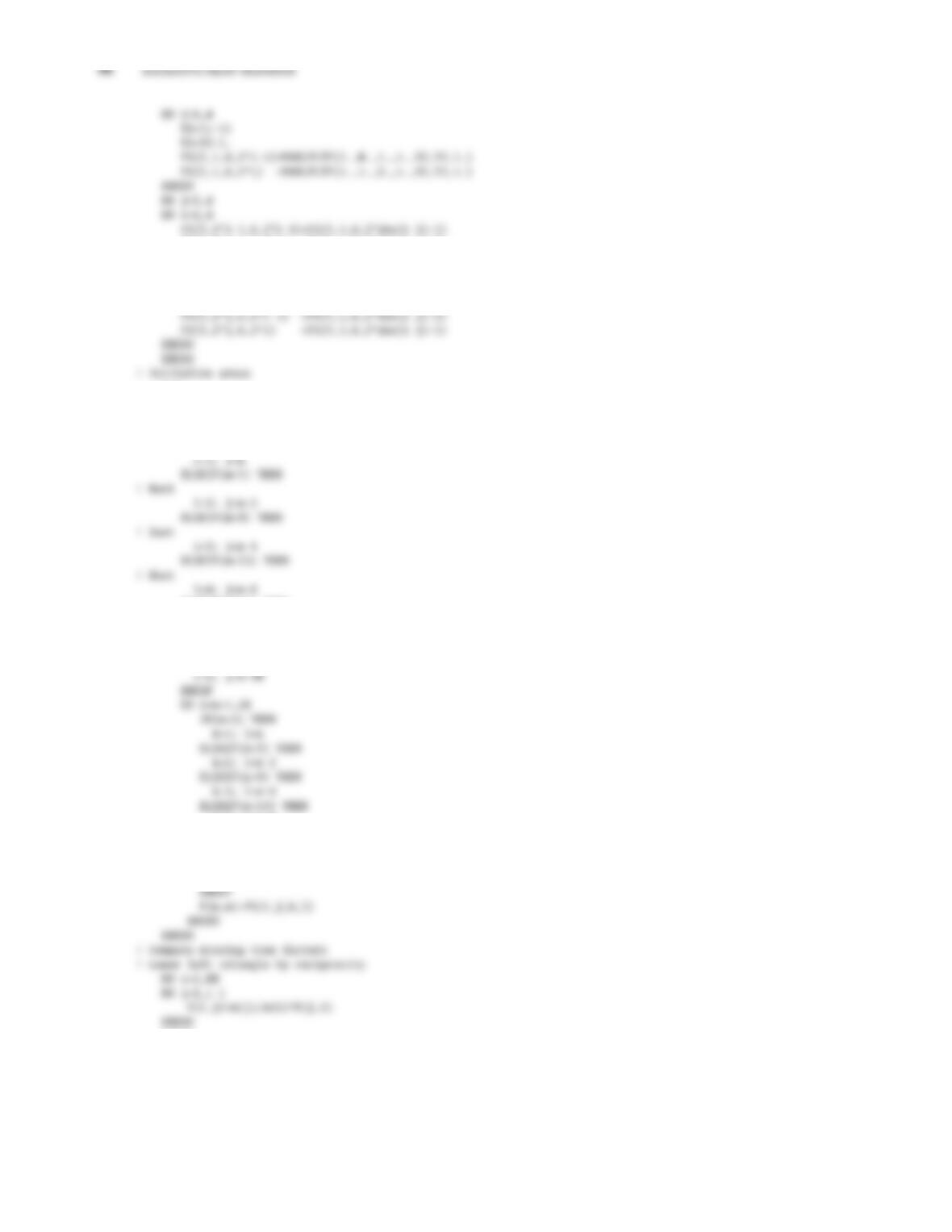FS(5,2*j-1,6,2*i) =FS(5,1,6,2*abs(i-j)+2)
ENDDO
ENDDO
DO j=1,4
DO i=1,4
A=1.
! Convert FS to 2D array; set surface emittances and temperatures/heat fluxes
DO m=1,28
IF(m<3) THEN
! Front
ELSEIF(m<21) THEN
! North
i=5; j=m-12
ELSE
! South
k=4; l=n-8
ELSEIF(n<21) THEN
k=5; l=n-12
ELSE
k=6; l=n-20
ENDDO
! Make sure summation rule is fulfilled
do i=1,NN
sum(i)=0.
do j=1,NN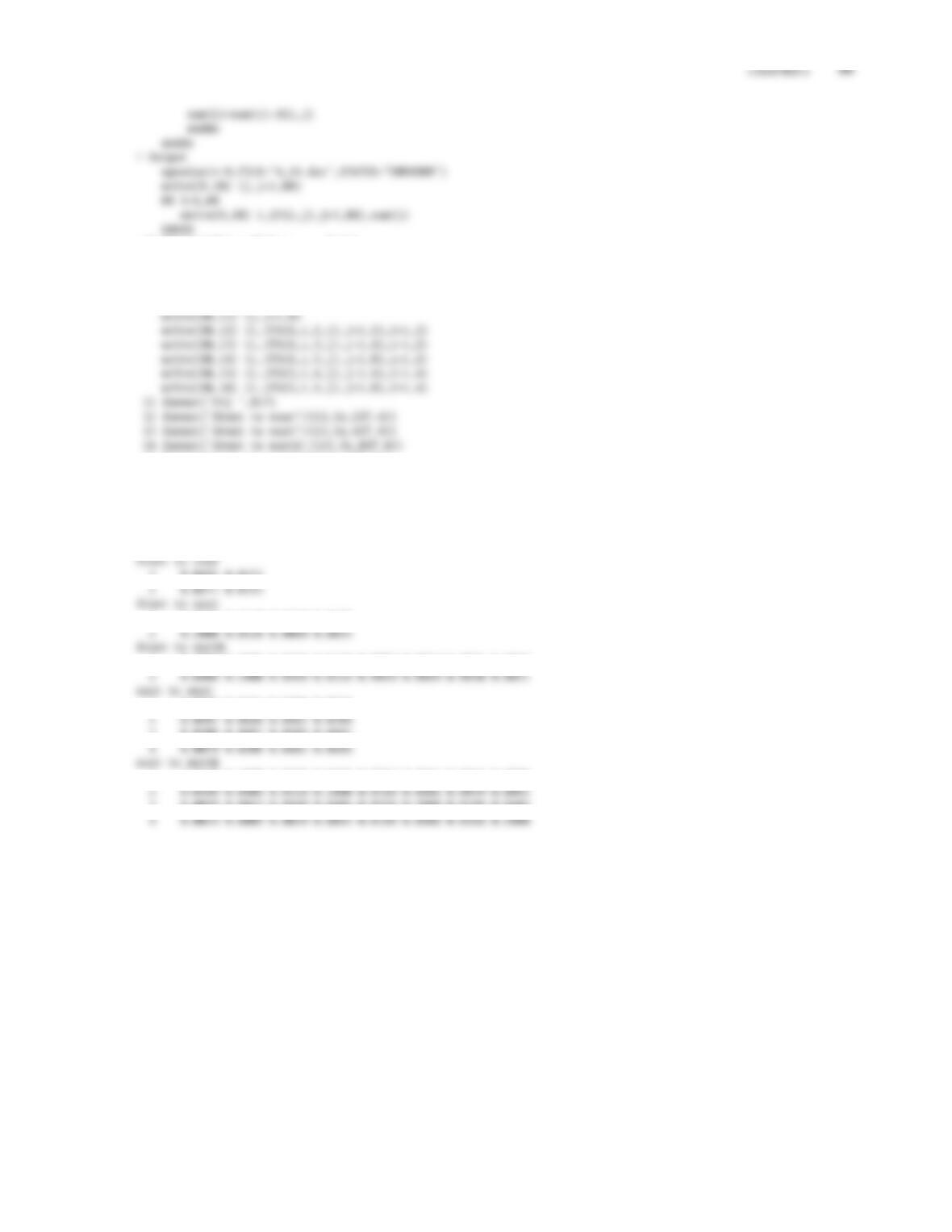10 format(’Fij ’,28i7,’ Sum’)
20 format(i3,3x,28f7.4,f8.4)
close(9)
! Printable output
open(unit=10,FILE=’4_22p.dat’,STATUS=’UNKNOWN’)
15 format(’east to west’/(i3,3x,4f7.4))
16 format(’east to north’/(i3,3x,8f7.4))
close(10)
stop
end
Fij 12345678
1 0.0191 0.0171
1 0.0328 0.0329 0.0159 0.0078
1 0.2000 0.0406 0.0328 0.0189 0.0089 0.0069 0.0035 0.0030
1 0.0686 0.0481 0.0206 0.0079
3 0.0206 0.0481 0.0686 0.0481
1 0.0328 0.2000 0.0189 0.0406 0.0059 0.0043 0.0019 0.0009
3 0.0059 0.0043 0.0189 0.0406 0.0328 0.2000 0.0189 0.0406

## Trusted by Thousands ofStudents

Here are what students say about us.